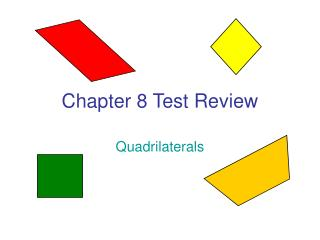DownloadDownload PresentationChapter 8 Test Review

# Chapter 8 Test Review

Download Presentation## Chapter 8 Test Review

- - - - - - - - - - - - - - - - - - - - - - - - - - - E N D - - - - - - - - - - - - - - - - - - - - - - - - - - -
##### Presentation Transcript

1. Chapter 8 Test Review Quadrilaterals

2. The sum of the measures of the interior angles of a convex regular polygon is 1080°. Classify the polygon by the number of sides. Octagon (8 sides)

3. What is the measure of each interior angle of a regular octagon? 1080  8 = 135°

4. 120° 97° x° 130° 150° Find the value of x. x + 120 + 97 + 130 + 150 + 90 = (6-4)(180) x = 133

5. 5x° 8x° 5x° Find the value of x. 8x + 5x + 5x = 360 x = 20

6. 10 8 m n - 3 Find the value of m and n in the parallelogram. n – 3 = 8 n = 11 m = 10

7. x + 9 2x + 4 What value of x makes the quadrilateral a parallelogram? 2x + 4 = x + 9 x = 5

8. y° 21° Find the values of x and y. x = 69 y = 21

9. 3x + 4 4y 6y - 10 4x - 5 Find the values of x and y. 3x + 4 = 4x – 5 x = 9 4y = 6y – 10 y = 5

10. 3x + 2 5x - 4 What value of x makes the quadrilateral a parallelogram? 3x + 2 = 5x – 4 x = 3

11. What is the most specific name for the polygon? Parallelogram

12. 125° 2x° 160° 110° x° 112° 147° Find the value of x. x + 160 + 2x + 125 + 110 + 112 + 147 = (7-2)(180) x = 82

13. 14 11 d + 4 c + 5 Find the values of c and d in the parallelogram. c + 5 = 11 c = 6 d + 4 = 14 d = 10

14. 18 (b + 16)° 103° a - 10 Find the values of a and b in the parallelogram. a -10 = 18 a = 28 b + 16 = 103 b = 87

15. In a regular nonagon, the exterior angles are all congruent. What is the measure of one of the exterior angles? 9x = 360 x = 40°

16. B A 20° C D ABCD is a kite.Find mB and mD. 90 + 20 + x + x = 360 mB = mD = 125°

17. What is the most specific name for the polygon? Quadrilateral RSTU has R, T, and U as right angles. RS = ST. Square

18. 19 in F G 16.5 in D E J H Find JH. (JH + 19)  2 = 16.5 JH = 14 in

19. E D 110° 25° F G DEFG is a trapezoid.Find mG and mE. mG = 70° mE = 155°